# The sum of divisors of $n$

Let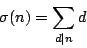denote the sum of the divisors of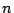.

G. Robin [ MR 86f:11069] showed that the Riemann Hypothesis is equivalent to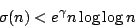for all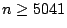, where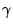is Euler's constant. That inequality does not leave much to spare, for Gronwall showed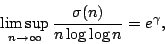and Robin showed unconditionally that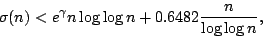for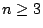.

J. Lagarias [ arXiv:math.NT/0008177] elaborated on Robin's work and showed that the Riemann Hypothesis is equivalent tofor all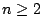, where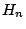is the harmonic number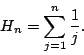By definition,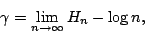so Lagarias' and Robin's inequalities are the same to leading order.

Back to the main index for The Riemann Hypothesis.